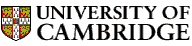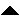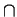Computer LaboratoryComputer Science Syllabus - Complexity TheoryComplexity TheoryNext: Databases Up: Easter Term 2006: Part Previous: Artificial Intelligence I   Contents

## Complexity Theory

Lecturer: Dr A.C. Norman

No. of lectures: 12

Prerequisite courses: Data Structures and Algorithms, Computation Theory

Aims

The aim of the course is to introduce the theory of computational complexity. The course will explain measures of the complexity of problems and of algorithms, based on time and space used on abstract models. Important complexity classes will be defined, and the notion of completeness established through a thorough study of NP-completeness. Applications to cryptographic protocols will be considered.

Lectures

• Algorithms and problems. Complexity of algorithms and of problems. Lower and upper bounds. Examples: sorting and travelling salesman.

• Time and space. Models of computation and measures of complexity. Time and space complexity on a Turing machine. Decidability and complexity.

• Time complexity. Time complexity classes. Polynomial time problems and algorithms. P and NP.

• Non-determinism. Non-deterministic machines. The class NP redefined. Non-deterministic algorithms for reachability and satisfiability.

• NP-completeness. Reductions and completeness. NP-completeness of satisfiability.

• More NP-complete problems. Graph-theoretic problems. Hamiltonian cycle and clique.

• More NP-complete problems. Sets, numbers and scheduling. Matching, set covering and bin packing.

• coNP. Validity of Boolean formulas and its completeness. NPcoNP. Primality and factorisation.

• Cryptographic complexity. One-way functions. The class UP.

• Space complexity. Deterministic and non-deterministic space complexity classes. The reachability method. Savitch's theorem.

• Hierarchy. The time and space hierarchy theorems and complete problems.

• Protocols. Interactive proofs. Zero knowledge.

Objectives

At the end of the course students should

• be able to analyse practical problems and classify them according to their complexity

• be familiar with the phenomenon of NP-completeness, and be able to identify problems that are NP-complete

• be aware of a variety of complexity classes and their interrelationships

• understand the role of complexity analysis in cryptographic protocols© 2005 University of Cambridge Computer Laboratory Please send any comments to pagemaster@cl.cam.ac.uk Page last updated on 11-Sep-2005 at 15:57 by Christine Northeast